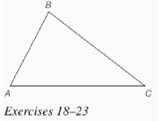Chapter 7.CR, Problem 18CR### Elementary Geometry for College St...

6th Edition
Daniel C. Alexander + 1 other
ISBN: 9781285195698

#### Solutions

Chapter
Section### Elementary Geometry for College St...

6th Edition
Daniel C. Alexander + 1 other
ISBN: 9781285195698
Textbook Problem
1 views

# In Review Exercises 18 to 23, use construction methods with the accompanying figure. Given: Δ A B CFind: The incenter

To determine

To find: The incenter of the triangle ABC.

Explanation

Given:

The triangle ABC is shown below.

Theorem used:

The three angle bisectors of the angles of a triangle are concurrent.

Calculation:

Consider the triangle ABC.

First draw the angular bisectors of A,B,C.

### Still sussing out bartleby?

Check out a sample textbook solution.

See a sample solution

#### The Solution to Your Study Problems

Bartleby provides explanations to thousands of textbook problems written by our experts, many with advanced degrees!

Get Started

#### Limits at Infinity Find the limit. 18. limxsin2x

Precalculus: Mathematics for Calculus (Standalone Book)

#### In Exercises 7-10, find the slope of the line shown in each figure. 8.

Applied Calculus for the Managerial, Life, and Social Sciences: A Brief Approach

#### In Problems 1-4, find the differential of each function.

Mathematical Applications for the Management, Life, and Social Sciences

#### Sometimes, Always, or Never: If for all n and both {an} and {cn} converge, then{bn} converges.

Study Guide for Stewart's Single Variable Calculus: Early Transcendentals, 8th

#### Minimize C=4x+y Subjected to 2x+y102x+3y24x+3y15x0,y0

Finite Mathematics for the Managerial, Life, and Social Sciences

#### Describe the general characteristics of the survey research design.

Research Methods for the Behavioral Sciences (MindTap Course List)